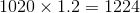# Basic Arithmetic : Creating Linear Equations with Part, Percent, and Whole

## Example Questions

### Example Question #2 : Percents And Decimals

What is the perimeter of a semicircle with an area of?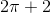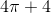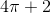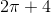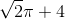Explanation:

This is a multi-step problem.  The perimeter of a semicircle is the sum of the circumference and diameter.  First, since we are given the area, we will need to find the radius.

For the area of a semicircle: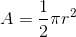Since the area is, substitute this into A to find radius r.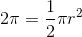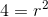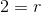Since the radius is 2, the diameter is 4.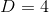The circumference for a semicircle is: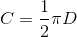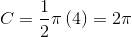The perimeter is the sum of the circumference and diameter:### Example Question #81 : Basic Arithmetic

What is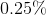of?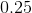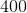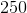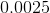Explanation:

Before multiplying, it's necessary to convert 0.25% to a decimal, which is 0.0025.

Multiply this 0.0025, with 100.

### Example Question #1 : Creating Linear Equations With Part, Percent, And Whole

What is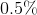of?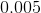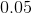Explanation:

Before multiplying, we can convert% into a decimal: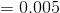.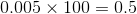### Example Question #11 : Percents And Decimals

What is the perimeter of a rectangle with an area of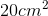, a length of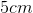, and a width of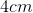?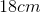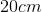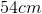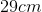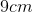Explanation:

Based on the information given in the question, we know that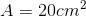. Since we also know the values of length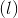and width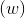, however, we don't need to use the area equation to solve forand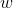, since the perimeter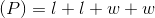.

Substituting in the known values ofand: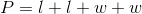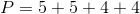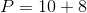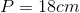### Example Question #1 : Creating Linear Equations With Part, Percent, And Whole

What is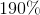of?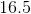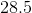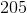Explanation:

Before we answer the question, we should convertinto a decimal:=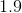.

We can then substitute this value into the original question: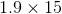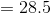### Example Question #11 : Percents And Decimals

Lucy is a real estate agent who is paid a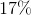commission on the value of each house she sells every month. She is also paid a base monthly rate of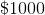as part of her salary. Ifrepresents the value of the houses she sells per month, which of the following equations would accurately predict how much money she makes per month?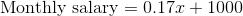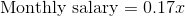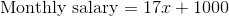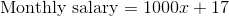Explanation:

To start, we need to convert the percent into a decimal.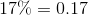Now, we know that Lucy receives 17% of the value of the homes she sells as her commission. Her commission can then be written as the expression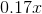.

Lucy is also paid \$1000 regardless of if she sells any houses or not that month. In a linear equation, this would be our y-intercept.

Putting the pieces of the salary together, we get the following equation:### Example Question #13 : How To Find Percentage

A school board plans to renovate one of its schools in order to make room for more people. After the renovation, the school's maximum capacity will increase by 20%. If the school's current maximum capacity is 1020 people, what will its maximum capacity be after the renovation?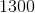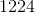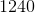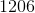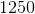Explanation:

A 20% increase is equal to 120% of the original value. To figure out how much more space the building will have if expanded 20%, you must multiply the existing maximum capacity (1020) by 120%. To do this, first divide 120 by 100.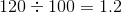Then, multiply the result times the existing maximum capacity.